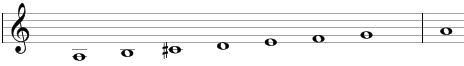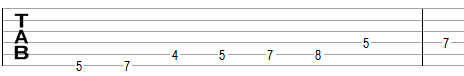# Major-minor scale

The major-minor scale is a secondary heptatonic scale that can be built using the following steps between notes: 1, 1, ½, 1, ½, 1, 1.

An example of the scale is A, B, C#, D, E, F, G. This example in shown below in traditional notation and in guitar tablature notation.This scale is called "major-minor" as it is essentially the natural minor scale with a raised (a major) third (the natural minor scale in the example above is A, B, C, D, E, F, G and the raised third is C#).

## Modes of the major-minor scale

The following are modes of the major-minor scale.

1. Major-minor, with steps 1, 1, ½, 1, ½, 1, 1 (A, B, C#, D, E, F, G in the example above).
2. Locrian with a sharp second, with steps 1, ½, 1, ½, 1, 1, 1 (B, C#, D, E, F, G, A).
3. Altered (Locrian with a flat fourth), with steps ½, 1, ½, 1, 1, 1, 1 (C#, D, E, F, G, A, B).
4. Melodic ascending minor, with steps 1, ½, 1, 1, 1, 1, ½ (D, E, F, G, A, B, C#).
5. Phrygian with a sharp sixth, with steps ½, 1, 1, 1, 1, ½, 1 (E, F, G, A, B, C#, D).
6. Lydian with a sharp fifth, with steps 1, 1, 1, 1, ½, 1, ½ (F, G, A, B, C#, D, E).
7. Lydian-Mixolydian, with steps 1, 1, 1, ½, 1, ½, 1 (G, A, B, C#, D, E, F).

## Three-note chords on the major-minor scale

The following are common triads built on the notes of the major-minor scale.

• On the root of the scale (on the tonic): major chord (e.g., A composed of A, C#, E), augmented chord (Aaug = A, C#, F), or suspended chords (Asus4 = A, D, E and Asus2 = A, B, E).
• On the second note (on the supertonic): dimished chord (Bdim = B, D, F).
• On the third note (on the mediant): augmented chord (C#aug = C#, F, A) or diminished chord (C#dim = C#, E, G).
• On the fourth note (on the subdominant): minor chord (Dm = D, F, A) or suspended chords (Dsus4 = D, G, A and Dsus2 = D, E, A).
• On the fifth note (on the dominant): minor chord (E = E, G, B) or suspended chord (Esus4 = E, A, B).
• On the sixth note (on the submediant): augmented chord (Faug = F, A, C#).
• On the seventh note (on the leading tone): major chord (G = G, B, D) or suspended chord (Gsus2 = G, A, D).

## Four-note chords on the major-minor scale

The following are seventh chords built on the notes of the major-minor scale.

• On the first note: dominant seventh chord (e.g., A7 composed of A, C#, E, G) or augmented seventh chord (A7#5 = A, C#, F, G).
• On the second note: half-diminished seventh chord (minor seventh chord with a flat fifth, Bm7b5 = B, D, F, A).
• On the third note: half-diminished seventh chord (C#m7b5 = C#, E, G, B), augmented seventh chord (C#7#5 = C#, F, A, B).
• On the fourth note: minor-major seventh chord (Dmmaj7 = D, F, A, C#).
• On the fifth note: minor seventh chord (Emin7 = E, G, B, D).
• On the sixth note: augmented major seventh chord (major seventh chord with a raised fifth, Fmaj7#5 = F, A, C#, E).
• On the seventh note: dominant seventh chord (G7 = G, B, D, F).

## Intervals on the major-minor scale

The major-minor scale is composed of the following intervals.

• A major second, e.g., the interval between A and B is equal to two semitones.
• A major third, the interval between A and C# is equal to four semitones.
• A perfect fourth, the interval between A and D is equal to five semitones.
• A perfect fifth, the interval between A and E is equal to seven semitones.
• A minor sixth, the interval between A and F is equal to eight semitones.
• A minor seventh, the interval between A and G is equal to ten semitones.

Scale, Scale (index)

### Filtered HTML

• Freelinking helps you easily create HTML links. Links take the form of [[indicator:target|Title]]. By default (no indicator): Click to view a local node.